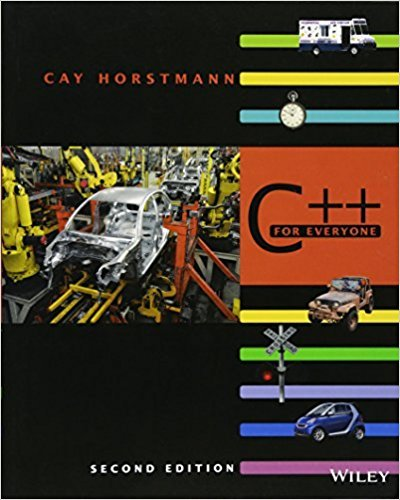×
Get Full Access to C++ For Everyone - 2 Edition - Chapter 7 - Problem R7.16
Get Full Access to C++ For Everyone - 2 Edition - Chapter 7 - Problem R7.16

×

# Consider this program segment:char a[] = "Mary had a little lamb";char* p = a;int countISBN: 9780470927137 356

## Solution for problem R7.16 Chapter 7

C++ for Everyone | 2nd Edition

• Textbook Solutions
• 2901 Step-by-step solutions solved by professors and subject experts
• Get 24/7 help from StudySoup virtual teaching assistantsC++ for Everyone | 2nd Edition

4 5 1 253 Reviews
17
5
Problem R7.16

Consider this program segment:char a[] = "Mary had a little lamb";char* p = a;int count = 0;while (*p != '\0'){ count++; while (*p != ' ' && *p != '\0') { p++; } while (*p == ' ') { p++; }}What is the value of count at the end of the outer while loop?

Step-by-Step Solution:
Step 1 of 3

Systems Wednesday, March 9, 22:43 PM • System ○ Definition: a domain of investigation ○ Thermodynamic System: the collection of matter or region of space ○ To define a system, must also define the environment or surroundings ○ Zero mathematical thickness of a system ○ The boundary is shared by both system and surroundings ○ Properties vary continuously across the surface of the boundary § There is no jump in properties across the boundary ○ Types: § Isolated □ A system that doesn’t allow transfer or mass or heat(work) to cross boundary □ Boundary is not free to move □ Rigid □ § Closed □ Cannot exchange mass (matter) across the boundary but can exchange heat or work □ □ § Adiabatic system □ Can exchange energy in the form of work but not heat or matter □ Work due to the expansion or compression of the boundary □ Virtually non existent in nature □

Step 2 of 3

Step 3 of 3

##### ISBN: 9780470927137

The answer to “Consider this program segment:char a[] = "Mary had a little lamb";char* p = a;int count = 0;while (*p != '\0'){ count++; while (*p != ' ' && *p != '\0') { p++; } while (*p == ' ') { p++; }}What is the value of count at the end of the outer while loop?” is broken down into a number of easy to follow steps, and 54 words. Since the solution to R7.16 from 7 chapter was answered, more than 263 students have viewed the full step-by-step answer. C++ for Everyone was written by and is associated to the ISBN: 9780470927137. This full solution covers the following key subjects: . This expansive textbook survival guide covers 10 chapters, and 515 solutions. This textbook survival guide was created for the textbook: C++ for Everyone , edition: 2. The full step-by-step solution to problem: R7.16 from chapter: 7 was answered by , our top Engineering and Tech solution expert on 03/08/18, 08:39PM.

## Discover and learn what students are asking

Statistics: Informed Decisions Using Data : Introduction to the Practice of Statistics
?A(n) ________ is a numerical summary of a sample. A(n) ________ is a numerical summary of a population.

Statistics: Informed Decisions Using Data : The Normal Probability Distribution
?Use the figure to answer the questions that follow. (a) What is ?? (b) What is ?? (c) Suppose that the area under the

Unlock Textbook Solution